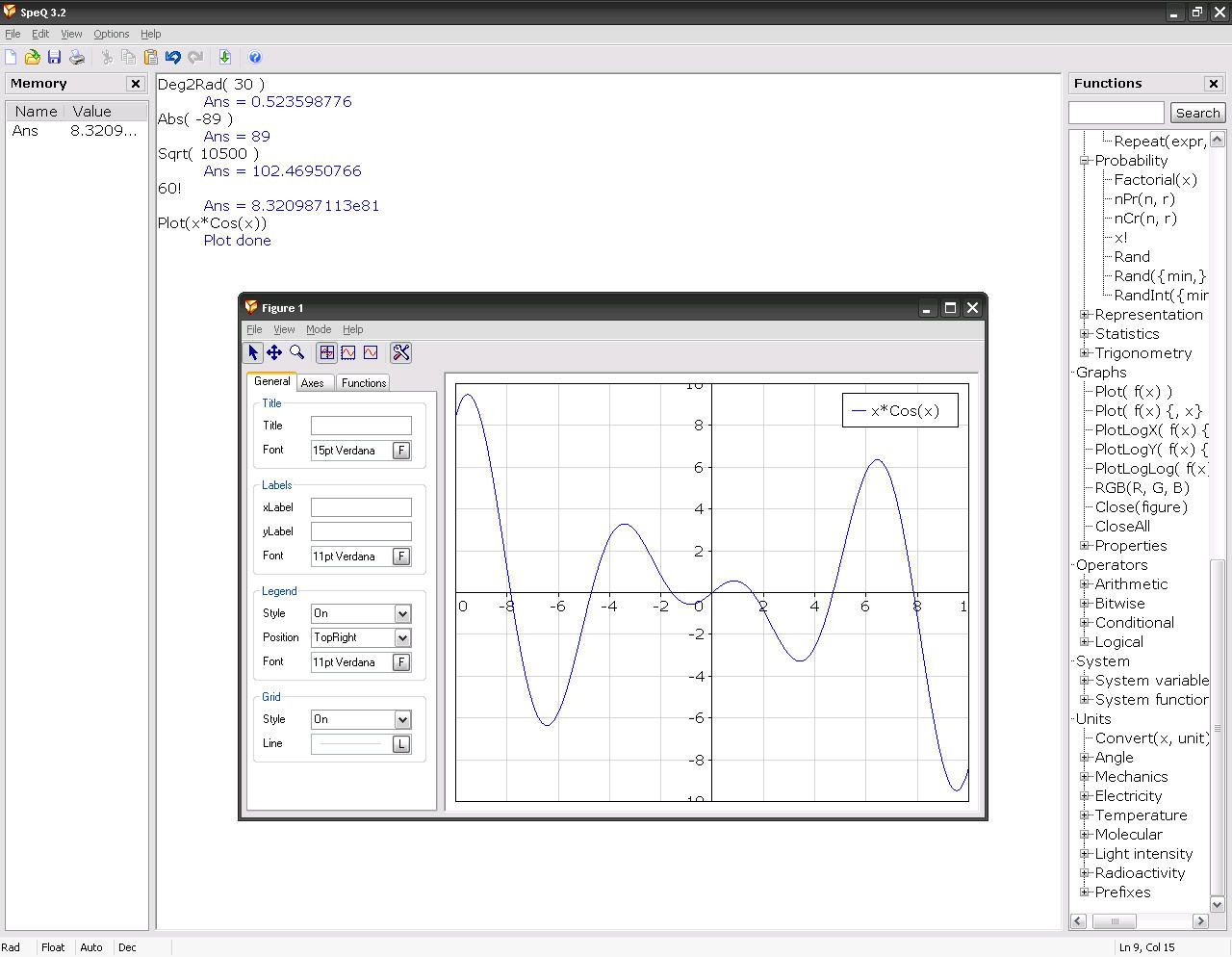SpeQ is a small, extensive mathematics program with a simple, intuitive interface. All calculations are entered in a sheet. In there you can freely add, edit and execute all calculations. You can define variables and functions, and plot graphs of your functions. You can save your calculations for later re-use.

SpeQ is written to be an advanced but very easy to use math program. It is small and fast and runs on every Windows computer, also from an USB-key or floppy disk. SpeQ is useful for simple, brief calculations as well as working out sheets with extensive variable definitions, functions and complex calculations.

SpeQ is usable for engineers, students and pupils or simply as replacement of the Windows Calculator.

SpeQ has the following features

Expression calculations with arithmetic, conditional, logical and bitwise operators
Around 100 built-in mathematical, physical and conditional constants
Around 60 built-in functions for Analysis, Arithmetic, Complex numbers, Hyperbolic, Integers, Logical, Probability, Numeral systems, Statistics, Trigonometry
Define your own variables and functions for use in calculations
Extensive support for units
Decimal, binary, hexadecimal and octal numeral systems
Calculations with lists
Complex number calculations
Colored workarea